In :
# %load ../../preconfig.py
%matplotlib inline
import matplotlib.pyplot as plt
import seaborn as sns
sns.set(color_codes=True)
plt.rcParams['axes.grid'] = False

#import numpy as np
#import pandas as pd
#import itertools

import logging
logger = logging.getLogger()


# 23 Minimum Spanning Trees¶

minimum-spanning-tree problem:
Given a connected, undirected graph $G = (V, E)$, and each edge $(u, v)$ has a weight $w(u, v)$.

Then we wish to find an acyclic subset $T \subseteq E$: $$\operatorname{argmin}_{T} \, w(T) = \displaystyle \sum_{(u, v) \in T} w(u, v)$$

We will use greedy algorithms to solve the minimum-spanning-tree problem, and we can prove that certain greedy strategies do yield a spanning tree with minimum weight.

### 23.1 Growing a minimum spanning tree¶

The generic method manages a set of edges $A$, maintaining the following loop invariant:
Prior to each iteration, $A$ is a subset of some minimum spanning tree.

We can add an edge safely to $A$ while maintaing the invariant, then we call such an edge a safe edge for $A$.

In :
plt.imshow(plt.imread('./res/generic.png'))

Out:
<matplotlib.image.AxesImage at 0x109293860>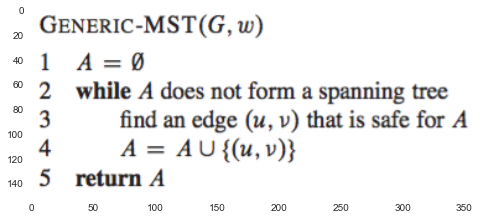#### rule for recognizing safe edges¶

define:

1. $\operatorname{cut}(S, V-S) \subset G$.

2. We say an edge $(u, v)$ crosses the cut $(S, V-S)$ if $u \in S, v \in V - S$.

3. We say a cut respects a set $A$ of edges if no dege in $A$ crosses the cut.

4. light edge: its weight is the minimum of any edge crosing the cut.

Our rule for recognizing safe edges is given by the following theorem:

##### Theorem 23.1¶

Let $G = (V, E)$ be a connected, undirected graph with a real-valued weight function $w$ defined on $E$. Let $A$ be a subset of $E$ that is included in some minimum spanning tree for $G$, let $(S, V - S)$ be any cut of $G$ that respects $A$, and let $(u, v)$ be a light edge crossing $(S, V - S)$. Then, edge $(u, v)$ is safe for $A$.

proof: construct.
details seen in textbook.

##### Corollary 23.2¶

Let $G = (V, E)$ be a connected, undirected graph with a real-valued weight function $w$ defined on $E$. Let $A$ be a subset of $E$ that is included in some minimum spanning tree for $G$, and let $C = (V_C, E_C)$ be a connected component (tree) in the forest $G_A = (V, A)$. If $(u, v)$ is a light edge connecting $C$ to some other component in $G_A$, then $(u, v)$ is safe for $A$.

In :
# exercise


### 23.2 The algorithms of Kruskal and Prim¶

These two algorithms each use a specific rule to determine a safe edge in line 3 of GENREIC-MST.

#### Kruskal's algorithm¶

The set of $A$ is a forest. The safe edge added to $A$ is always a least-weight edge in the graph that connects two distinct components.

In :
plt.imshow(plt.imread('./res/kru.png'))

Out:
<matplotlib.image.AxesImage at 0x109cc0f98>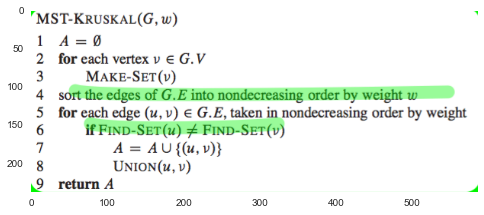In :
plt.figure(figsize=(8,12))

Out:
<matplotlib.image.AxesImage at 0x10b75ecf8>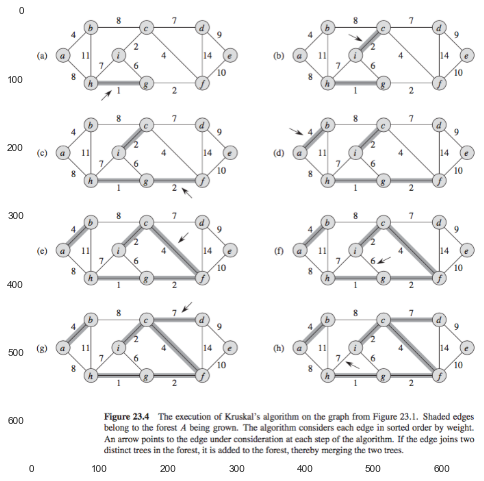#### Prim's algorithm¶

The set $A$ forms a single tree. The safe edge added to $A$ is always a least-weight edge connecting the tree to a vertex not in the tree.

In order to implement Prim’s algorithm efficiently, we need a fast way to select a new edge to add to the tree formed by the edges in $A$:
all vertices that are not in the tree reside in a min-priority queue $Q$ based on a $key$ attribute. For each vertex $v$, the attribute $v.\text{key}$ is the minimum weight of any edge connecting $v$ to a vertex in the tree.

$$A = \left \{(v, v.\pi) : v \in V - \{r\} - Q \right \}$$
In :
plt.imshow(plt.imread('./res/prim.png'))

Out:
<matplotlib.image.AxesImage at 0x10c3e53c8>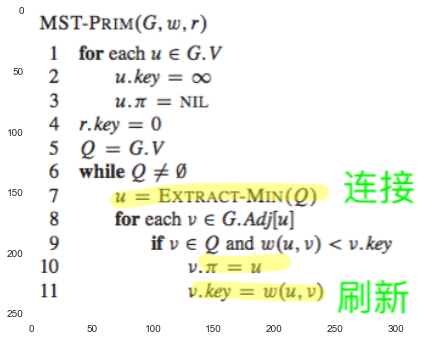In :
plt.figure(figsize=(8,12))

<matplotlib.image.AxesImage at 0x10cc22b00>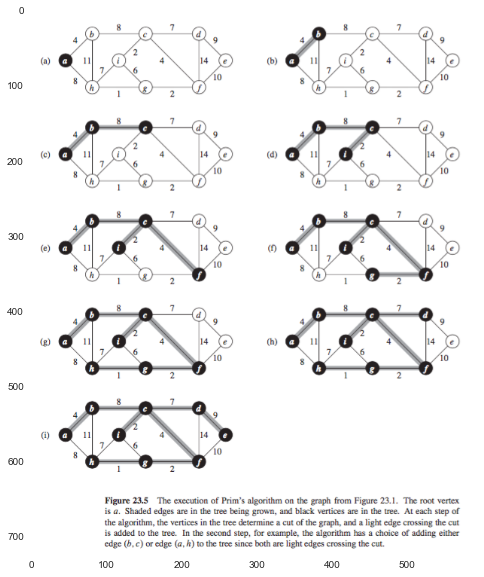# Exercise